Test: Geometry- 1

# Test: Geometry- 1

Test Description

## 15 Questions MCQ Test CSAT Preparation for UPSC CSE | Test: Geometry- 1

Test: Geometry- 1 for Quant 2022 is part of CSAT Preparation for UPSC CSE preparation. The Test: Geometry- 1 questions and answers have been prepared according to the Quant exam syllabus.The Test: Geometry- 1 MCQs are made for Quant 2022 Exam. Find important definitions, questions, notes, meanings, examples, exercises, MCQs and online tests for Test: Geometry- 1 below.
Solutions of Test: Geometry- 1 questions in English are available as part of our CSAT Preparation for UPSC CSE for Quant & Test: Geometry- 1 solutions in Hindi for CSAT Preparation for UPSC CSE course. Download more important topics, notes, lectures and mock test series for Quant Exam by signing up for free. Attempt Test: Geometry- 1 | 15 questions in 30 minutes | Mock test for Quant preparation | Free important questions MCQ to study CSAT Preparation for UPSC CSE for Quant Exam | Download free PDF with solutions
 1 Crore+ students have signed up on EduRev. Have you?
Test: Geometry- 1 - Question 1

### A vertical stick 20 m long casts a shadow 10 m long on the ground. At the same time, a tower casts a shadow 50 m long on the ground. Find the height of the tower.

Detailed Solution for Test: Geometry- 1 - Question 1
• When the length of stick = 20 m, then length of shadow = 10 m i.e. in this case length = 2 * shadow
• With the same angle of inclination of the sun, the length of the tower that casts a shadow of 50 m is: 2 * 50m = 100m
⇒ Height of tower = 100 m
Test: Geometry- 1 - Question 2

### The area of similar triangles, ABC and DEF are 144cm2 and 81 cm2 respectively. If the longest side of the larger △ABC be 36 cm, then the longest side of the smaller △DEF is:

Detailed Solution for Test: Geometry- 1 - Question 2

For similar triangles

⇒ (Ratio of sides) = Ratio of areas

Then as per question = (36/x)= 141/81

Let the longest side of ΔDEF = x

⇒ 36/x = 12/9

⇒ x = 27cm

Test: Geometry- 1 - Question 3

### Find the value of x in the figure, if it is given that AC and BD are diameters of the circle.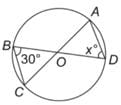Detailed Solution for Test: Geometry- 1 - Question 3
• The triangle BOC is an isosceles triangle with sides OB and OC both being equal as they are the radii of the circle. Hence, the angle OBC = angle OCB = 30°.
• Hence, the third angle of the triangle BOC i.e. Angle BOC would be equal to 120°.
⇒ BOC = AOD = 120°
• Also, in the isosceles triangle DOA:
Angle ODA = Angle DAO = x = 30°
Test: Geometry- 1 - Question 4

Find the value of x in the given figure.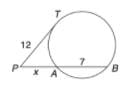Detailed Solution for Test: Geometry- 1 - Question 4

By the rule of tangents, we get:

⇒ 122 = (x + 7)x
⇒ 144 = x2 + 7x
⇒ x2 + 7x – 144 = 0
⇒ x2 +16x – 9x –144 = 0
⇒ x(x + 16) – 9(x + 16) = 0
⇒ x = 9 or –16

–16 can’t be the length, hence this value is discarded. Thus, x = 9

Test: Geometry- 1 - Question 5

Find the value of x in the given figure.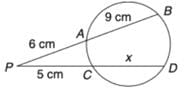Detailed Solution for Test: Geometry- 1 - Question 5

By the rule of chords, cutting externally, we get:

(9 + 6) * 6 = (5 + x) * 5
90 = 25 + 5x
5x = 65
x = 13 cm

Test: Geometry- 1 - Question 6

In the given figure ㄥPAB = 25º. AB is the diameter. Find ㄥTPA.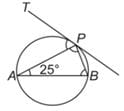Detailed Solution for Test: Geometry- 1 - Question 6
• ㄥAPB = 90° (angle in a semicircle = 90°)
• ㄥPBA = 180 – (90 + 25) = 65°
• ㄥTPA = ㄥPBA (the angle that a chord makes with the tangent, is subtended by the chord on the circumference in the alternate segment) = 65°

Note: This is also called the Alternate Segment Theorem.

Test: Geometry- 1 - Question 7

In the figure, AB is parallel to CD and RD || SL || TM || AN, and BR : RS : ST : TA = 3 : 5 : 2 : 7. If it is known that CN = 1.333 BR. Find the ratio of BF : FG : GH : HI : IC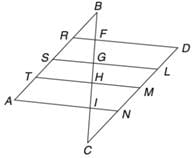Detailed Solution for Test: Geometry- 1 - Question 7
• Since the lines, AB and CD are parallel to each other, and the lines RD and AN are parallel, it means that the triangles RBF and NCI are similar to each other. Since the ratio of CN : BR = 1.333, if we take BR as 3, we will get CN as 4.
• This means that the ratio of BF : CI would also be 3 : 4.
Also, the ratio of BR : RS : ST : TA = BF : FG : GH : HI = 3 : 5 : 2 : 7 (given).

Hence, the correct answer is 3 : 5 : 2 : 7 : 4

Test: Geometry- 1 - Question 8

In the following figure, it is given that O is the centre of the circle and ㄥAOC = 140°. Find ㄥABC.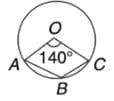Detailed Solution for Test: Geometry- 1 - Question 8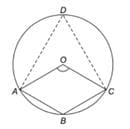∠AOC of minor sector = 140°
∠AOC of major sector=360° - 140° = 220°
Theorem: The angle subtended at the centre is twice the angle formed at the circumference of the circle.
Hence,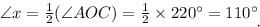∴ The measure of ∠x = 110°

Test: Geometry- 1 - Question 9

In the figure below, PQ = QS, QR = RS and angle SRQ = 100°. How many degrees is angle QPS?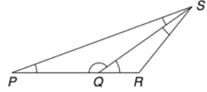Detailed Solution for Test: Geometry- 1 - Question 9

In ΔQRS, QR = RS
⇒ ㄥRQS = ㄥRSQ (because angles opposite to equal sides are equal).
Thus:

ㄥRQS + ㄥRSQ = 180° - 100° = 80°
ㄥRQS = ㄥRSQ = 40°
ㄥPQS = 180° – 40° = 140°  (sum of angles on a line = 180°)

Then again, ㄥQPS = ㄥQSP (since angles opposite to equal sides are equal)

ㄥQPS + ㄥQSP = 180° – 140° = 40°
ㄥQPS = ㄥQSP = 20°

Test: Geometry- 1 - Question 10

In the given figure, AD is the bisector of ΔBAC, AB = 6 cm, AC = 5 cm and BD = 3 cm. Find DC. It is given that ∠ABD = ∠ACD.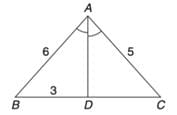Detailed Solution for Test: Geometry- 1 - Question 10

We know that the internal bisector of angle of a triangle divides the opposite side internally in the ratio of the sides containing the angle.
Hence:

AB/BD = AC/CD
6/3 = 5/CD
CD = 2.5 cm

Test: Geometry- 1 - Question 11

In the given figure, straight line AB and CD intersect at O. IF ∠δ =3∠v, then ∠v = ?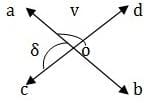Detailed Solution for Test: Geometry- 1 - Question 11

COD is a  straight line
∴ ∠δ + ∠v =180⁰ ⇒ 3v +v =180 ⇒ 4v = 180 ⇒ v =45⁰.

Test: Geometry- 1 - Question 12

In a triangle ABC, the incentre is at 0. If ㄥBOC = 100°, find ㄥBAC.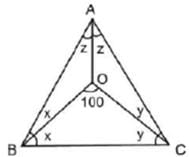Detailed Solution for Test: Geometry- 1 - Question 12

In ∠BOC

⇒ x + y = 80°

⇒ 2x + 2y. = 160°

Also, 2x + 2y + 2z = 180°

⇒ 160° + 2z = 180°

⇒ ∠BAC = 2z = 20°

Test: Geometry- 1 - Question 13

A rectangular enclosure 40 m x 36 m has a horse tethered to a corner with a rope of 14 m in length. What is the ratio of the respective areas it can graze if it is outside the enclosure and if it is inside the enclosure?

Detailed Solution for Test: Geometry- 1 - Question 13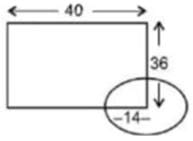• Imagine a circle on the corner of the rectangle
• 3 quarters of the circle lie outside the rectangle and 1 quarter lies inside.
• Hence, Required ratio = 3 : 1
Test: Geometry- 1 - Question 14

Read the passage below and solve the questions based on it.
The area of a square is equal to the area of a rectangle. Moreover, the perimeter of the square is also equal to the perimeter of the rectangle.

Q. The length of the rectangle is equal to the:

Detailed Solution for Test: Geometry- 1 - Question 14

This is possible only when both the length and breadth of the rectangle are equal to the side of the square.

Test: Geometry- 1 - Question 15

A quadrilateral is inscribed in a circle. If an angle is inscribed in each of the four segments outside the quadrilateral, then what is the sum of these four angles?

Detailed Solution for Test: Geometry- 1 - Question 15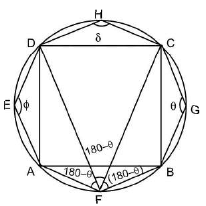Required sum = θ + 180° - θ + 180° - θ + 180° - θ + θ + 8 = 540°

## CSAT Preparation for UPSC CSE

72 videos|64 docs|92 tests
 Use Code STAYHOME200 and get INR 200 additional OFF Use Coupon Code
Information about Test: Geometry- 1 Page
In this test you can find the Exam questions for Test: Geometry- 1 solved & explained in the simplest way possible. Besides giving Questions and answers for Test: Geometry- 1, EduRev gives you an ample number of Online tests for practice

## CSAT Preparation for UPSC CSE

72 videos|64 docs|92 tests